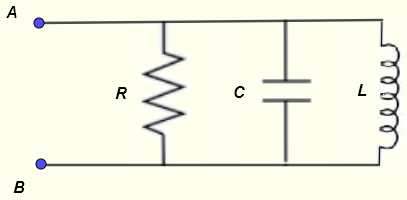# Parallel RLC circuit Impedance Calculator

A calculator to calculate the equivalent impedance of a resistor, a capacitor and and inductor in parallel. The calculator gives the impedance as a complex numbers in standard form , its modulus and argument which may be used to write the impedance in exponential and polar forms.

  

## Formulae for Parallel RLC Circuit Used in Calculator and their Units

We first give the formulas used in the parallel RLC calculator and the proof of these formulas is presented in the bottom part of the page.Let $f$ be the frequency, in Hertz, of the source voltage supplying the circuit.
and define the following parameters used in the calculations
$\omega = 2 \pi f$ , angular frequency in rad/s
$X_C = 1 / (\omega C)$ , the capacitor reactance in ohms $(\Omega)$
$X_L = \omega L$ , the inductor reactance in ohms $(\Omega)$
Let $Z$ be the equivalent impedance to the parallel RLC circuit shown above and write it in complex form as follows
$Z = r e^{j \theta}$
The formulae for the modulus $r$ and argument $\theta$ are given by

Modulus: $|Z| = r = \dfrac{1}{\sqrt { \left(\dfrac{1}{R}\right)^2 + \left(\dfrac{1}{{X_C}} - \dfrac{1}{ X_L} \right)^2}}$ in ohms $(\Omega)$

Argument: $\theta = \arctan \left(\dfrac{R}{X_L}-\dfrac{R}{X_C} \right)$ in radians or degrees

## Use of the calculator

Enter the resistance, the capacitance, the inductance and the frequency as positive real numbers with the given units then press "calculate".

 Resistance R = 50 mΩ Ω KΩ MΩ Capacitance C = 15 pF nF μF mF F Inductance L = 20 μH mH H Frequency f = 1.5 GHz MHz kH Hz mHz

## Proofs of the Formulae for The Parallel RLC Circuit

Let
$Z_R = R$ , $Z_C = \dfrac{1}{j \omega C}$ , $Z_L = j \omega L$
Apply the rule of impedances of a parallel circuits to find the equivalent impedance $Z$ as follows
$\dfrac{1}{Z} = \dfrac{1}{Z_R} + \dfrac{1}{Z_C} + \dfrac{1}{Z_L}$

$= \dfrac{1}{R} + \dfrac{1}{\dfrac{1}{j \omega C}} + \dfrac{1}{j \omega L}$
Let
$X_L = \omega L$ and $X_C = \dfrac{1}{\omega C}$
and rewrite the above as
$\dfrac{1}{Z} = \dfrac{1}{R} + \dfrac{1}{\dfrac{X_C}{j}} + \dfrac{1}{j X_L}$

$\dfrac{1}{Z} = \dfrac{1}{R} + \dfrac{j}{{X_C}} - j \dfrac{1}{ X_L}$

$\dfrac{1}{Z} = \dfrac{1}{R} + j (\dfrac{1}{{X_C}} - \dfrac{1}{ X_L} )$
The Modulus $\rho$ of the above complex number is given by
$\rho = \sqrt { \left(\dfrac{1}{R}\right)^2 + \left(\dfrac{1}{{X_C}} - \dfrac{1}{ X_L} \right)^2}$
and its argument $\alpha$ is given by
$\alpha = \arctan \left(\dfrac{\dfrac{1}{{X_C}} - \dfrac{1}{ X_L}}{\dfrac{1}{R}} \right)$
rearrange
$\alpha = \arctan \left(\dfrac{R}{X_C}-\dfrac{R}{X_L} \right)$
We now use the exponential form of complex number to write
$\dfrac{1}{Z} = \rho e^{j\alpha}$
We now write the equivalent impedance $Z$ as a complex number in exponential form by taking the reciprocal of the above
$Z = \dfrac{1}{\rho} e^{-j \alpha}$
Writing $Z$ as $Z = r e^{j\theta}$, we have
the modulus of $Z$ as
$r = 1/\rho = \dfrac{1}{\sqrt { \left(\dfrac{1}{R}\right)^2 + \left(\dfrac{1}{{X_C}} - \dfrac{1}{ X_L} \right)^2}}$
and the argument of $Z$ as
$\theta = \arctan \left(\dfrac{R}{X_L}-\dfrac{R}{X_C} \right)$

## Numerical Example Using the Above Formulas

$f = 1.5 \; kHz$ , $C = 15 \; \mu F$ , $L = 20 \; mH$ and $R = 50 \; \Omega$
$X_L = \omega L = 2 \pi f L = 2 \pi 1.5 \times 10^3 \times 20 10^{-3 } = 188.50$
$X_C = \dfrac{1}{\omega C} = \dfrac{1}{ 2\pi f C} = \dfrac{1}{ 2\pi 1.5 \times 10^3 \times 15 10^{-6}} = 7.07$
Modulus: $\dfrac{1}{\sqrt { \left(\dfrac{1}{R}\right)^2 + \left(\dfrac{1}{{X_C}} - \dfrac{1}{ X_L} \right)^2}}$

$= \dfrac{1}{\sqrt { \left(\dfrac{1}{50}\right)^2 + \left(\dfrac{1}{{7.07}} - \dfrac{1}{ 188.50} \right)^2}}$
$= 7.27$
Argument: $\arctan \left(\dfrac{R}{X_L}-\dfrac{R}{X_C} \right)$
$= \arctan \left(\dfrac{50}{188.50}-\dfrac{50}{7.07} \right)$
$= - 81.64^{\circ}$
You may input the given values in the calculator and check the results.